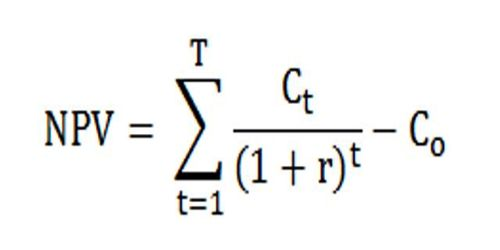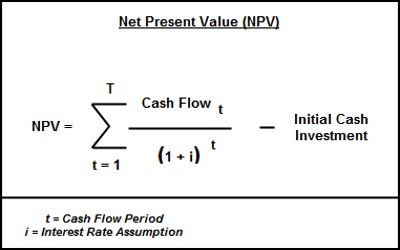# Net Present Value (NPV)

Net Present Value (NPV) is the difference between the present value of cash inflows and the present value of cash outflows. NPV is used in capital budgeting to analyze the profitability of an investment or project.

NPV is used to evaluate an investment assessment and give company management a plain way to tell if the investment will add value to the company. Naturally, if an investment has a positive net present value, it will add value to the company and advantage company shareholders.

The following is the formula for calculating NPV:

The formula for NPV is:

NPV = (Cash inflows from investment) – (cash outflows or costs of investment)or, NPV = TƩt=1 [Ct (1 + r)t – C0]

Where;

Ct = net cash inflow during the period,

C0 = initial investment,

r = discount rate,

and t = number of time periods.

In case of standalone projects, accept a project only if it’s NPV is positive, reject it if its NPV is negative, and stay indifferent between accepting and rejecting if NPV is zero. In case of mutually exclusive projects (i.e. competing projects), accept the project with higher NPV.